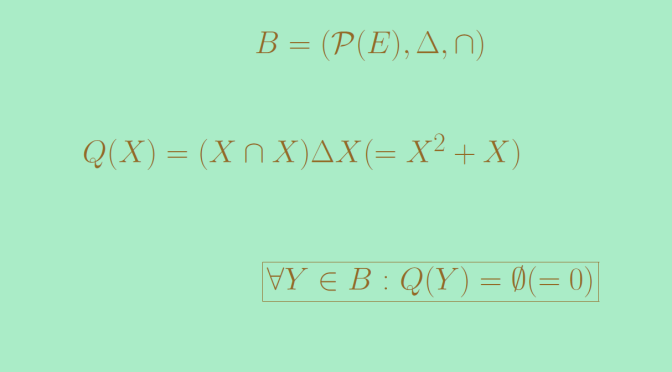# On polynomials having more roots than their degree

Let’s consider a polynomial of degree $$q \ge 1$$ over a field $$K$$. It is well known that the sum of the multiplicities of the roots of $$P$$ is less or equal to $$q$$.

The result remains for polynomials over an integral domain. What is happening for polynomials over a commutative ring?

## A polynomial of degree 2 having 4 roots

In this counterexample, we take for $$A=\mathbb{Z}/12\mathbb{Z}$$ the quotient ring of integers modulo $$12$$ and consider the polynomial $$P(X) = X^2 -4$$.
Obviously $$x_1=2$$ and $$x_2=-2=10$$ are roots of $$P$$. However that’s not the only ones! In particular, we also have:

• $$P(8)=64-4=5 \cdot 12=0$$
• $$P(10)=100-4=8 \cdot 12=0$$

So $$x_3=8$$ and $$x_4=10$$ are two other roots of $$P$$.
As expected through the introduction of the topic, $$A=\mathbb{Z}/12\mathbb{Z}$$ is not entire: $$3 \cdot 4 = 12 = 0$$ in $$A$$.

We also notice that $$P$$ has several factorizations. In particular:
$P(X)=X^2-4=(X-2)(X-10)=(X-8)(X-10)$

## A polynomial of degree 2 having an infinite number of roots

For this counterexample, let’s pick-up an infinite set $$E$$ and consider the power set $$B=\mathcal{P}(E)$$. On $$B$$, we can define two operations. The first one $$\Delta$$ is the symmetric difference and the second one $$\cap$$ is the intersection.

$$(B,\Delta, \cap)$$ is a commutative ring. The proof is left to the reader. The empty set $$\emptyset$$ is the unit element for $$\Delta$$ (our ring addition) while $$E$$ is the unit element for $$\cap$$ (our ring multiplication). $$B$$ is not entire as we can see by considering an element $$e \in E$$ and the subsets $$R=\{e\}$$ and $$\displaystyle S=\overline{\{e\}}$$: we have $$R \cap S= \emptyset$$.

We now look at the polynomial:
$Q(X) = (X \cap X) \Delta X$
$$Q(X)$$ would just be $$X^2+X$$ if we would have write $$\Delta$$ as $$+$$ and $$\cap$$ as $$\times$$.

Now for all $$T \in B=\mathcal{P}(E)$$ we have $$T \cap T = T$$ and $$T \Delta T = \emptyset$$. Hence all elements of $$B$$ are roots of $$Q$$. However $$Q$$ is not the zero polynomial.